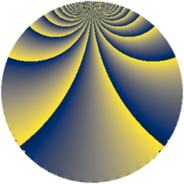# Properties

 Label 1815.2.jLevel $1815$ Weight $2$ Character orbit 1815.j Rep. character $\chi_{1815}(967,\cdot)$ Character field $\Q(\zeta_{4})$ Dimension $216$ Sturm bound $528$

# Related objects

## Defining parameters

 Level: $$N$$ $$=$$ $$1815 = 3 \cdot 5 \cdot 11^{2}$$ Weight: $$k$$ $$=$$ $$2$$ Character orbit: $$[\chi]$$ $$=$$ 1815.j (of order $$4$$ and degree $$2$$) Character conductor: $$\operatorname{cond}(\chi)$$ $$=$$ $$55$$ Character field: $$\Q(i)$$ Sturm bound: $$528$$

## Dimensions

The following table gives the dimensions of various subspaces of $$M_{2}(1815, [\chi])$$.

Total New Old
Modular forms 576 216 360
Cusp forms 480 216 264
Eisenstein series 96 0 96

## Trace form

 $$216q - 8q^{5} + O(q^{10})$$ $$216q - 8q^{5} - 16q^{12} + 8q^{15} - 248q^{16} + 40q^{20} + 32q^{23} + 8q^{25} - 16q^{26} + 16q^{31} - 216q^{36} + 8q^{37} + 56q^{38} - 8q^{42} - 32q^{48} - 24q^{53} - 176q^{56} - 32q^{60} + 48q^{67} - 8q^{70} + 64q^{71} - 16q^{75} + 48q^{78} + 160q^{80} - 216q^{81} + 96q^{82} - 96q^{86} + 48q^{91} - 40q^{92} + 48q^{93} - 88q^{97} + O(q^{100})$$

## Decomposition of $$S_{2}^{\mathrm{new}}(1815, [\chi])$$ into newform subspaces

The newforms in this space have not yet been added to the LMFDB.

## Decomposition of $$S_{2}^{\mathrm{old}}(1815, [\chi])$$ into lower level spaces

$$S_{2}^{\mathrm{old}}(1815, [\chi]) \cong$$ $$S_{2}^{\mathrm{new}}(55, [\chi])$$$$^{\oplus 4}$$$$\oplus$$$$S_{2}^{\mathrm{new}}(165, [\chi])$$$$^{\oplus 2}$$$$\oplus$$$$S_{2}^{\mathrm{new}}(605, [\chi])$$$$^{\oplus 2}$$